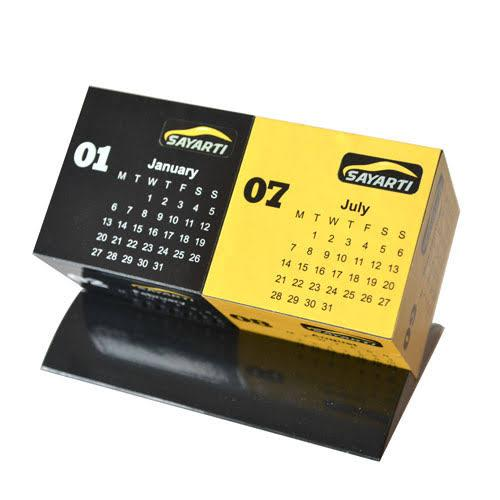# What Do You Know About Two-cube Calendar?

Approved & Edited by ProProfs Editorial Team
At ProProfs Quizzes, our dedicated in-house team of experts takes pride in their work. With a sharp eye for detail, they meticulously review each quiz. This ensures that every quiz, taken by over 100 million users, meets our standards of accuracy, clarity, and engagement.
A
Community Contributor
Total Contribution - 145 | Total attempts - 37,893
Questions: 10 | Attempts: 128SettingsSharpen your exploitative skill with this quiz on two-cube calender. In two-cube calendar, two cube-shaped figures are examined. These figures have faces tagged by numbers 0 through 9, and each of these would have one digit. Using simple mathematical skills, the two cubes are read in determination of dates. Are you sure you are in the know?

• 1.

### What is the total number of possible outcomes in the calendar?

• A.

14

• B.

13

• C.

12

• D.

11

D. 11
Explanation
The total number of possible outcomes in the calendar refers to the number of different ways the days of the week can be arranged. In a standard calendar, there are 7 days of the week. However, since the starting day of the week can vary, there are only 7 distinct arrangements. Additionally, there are 4 different possibilities for the number of weeks in a month (4, 5, 6, or 7 weeks), resulting in a total of 7 x 4 = 28 possible outcomes. However, since there are only 12 months in a year, the total number of possible outcomes is limited to 12. Therefore, the correct answer is 12.

Rate this question:

• 2.

### How many characters make up the set of letters often used in the calendar?

• A.

12 characters

• B.

18 characters

• C.

28 characters

• D.

10 characters

B. 18 characters
Explanation
The set of letters often used in the calendar includes the 12 letters representing the months (January to December) and the 6 letters representing the days of the week (Sunday to Saturday). Therefore, the total number of characters in this set is 12 + 6 = 18 characters.

Rate this question:

• 3.

### In the set of cubes, what does 6 double as?

• A.

18

• B.

12

• C.

9

• D.

7

C. 9
Explanation
In the set of cubes, the number 6 doubles as 9. This means that if you were to multiply 6 by 2, the result would be 9.

Rate this question:

• 4.

### How many faces of the cubes have numbers 3, 4, 5 on them?

• A.

4 faces

• B.

9 faces

• C.

7 faces

• D.

3 faces

D. 3 faces
Explanation
A cube has 6 faces in total. Since the numbers 3, 4, and 5 are mentioned on the faces, it is clear that these three faces have those numbers. Therefore, the correct answer is 3 faces.

Rate this question:

• 5.

### When was the problem of two-cube calendar first discussed in a publication?

• A.

1995

• B.

1994

• C.

1993

• D.

1992

D. 1992
Explanation
The problem of the two-cube calendar was first discussed in a publication in 1992.

Rate this question:

• 6.

### On how many faces is the solution to the two-cube calendar established?

• A.

31 faces

• B.

30 faces

• C.

3 faces

• D.

7 faces

A. 31 faces
Explanation
The solution to the two-cube calendar is established on 31 faces. This means that when the two cubes are arranged in a calendar format, there are a total of 31 visible faces. Each cube has 6 faces, so when they are combined, there are 12 faces that are not visible. Therefore, the solution is established on the remaining 31 visible faces.

Rate this question:

• 7.

### Which day of the month does the front faces of the two cubes indicate?

• A.

23rd day

• B.

15th day

• C.

20th day

• D.

25th day

D. 25th day
Explanation
The front faces of the two cubes indicate the 25th day of the month.

Rate this question:

• 8.

### When did Singleton's patent of the calendar lapse?

• A.

1965

• B.

1966

• C.

1967

• D.

1968

A. 1965
Explanation
Singleton's patent of the calendar lapsed in 1965.

Rate this question:

• 9.

### Following Gadner's receipt of an official letter, in which year was the patent of the calendar awarded?

• A.

1956

• B.

1957

• C.

1958

• D.

1959

B. 1957
Explanation
The correct answer is 1957 because the question states that Gardner received an official letter in which year the patent of the calendar was awarded. Since the answer options are in chronological order, we can infer that the patent was awarded in the year after Gardner received the letter, which is 1957.

Rate this question:

• 10.

### In the solution of the two-cube calendar problem, p is the inverse of what?

• A.

T

• B.

D

• C.

U

• D.

QBack to top